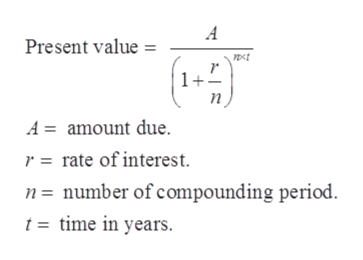# Find the present value of \$30,000 due in 6 years at an interest rate of 8%/year compounded monthly.

Question
3 views

Find the present value of \$30,000 due in 6 years at an interest rate of 8%/year compounded monthly.

check_circle

Step 1

Given:

Find the present value of \$30,000 due in 6 years at an interest rate of 8%/year compounded monthly.

Step 2

Formula used:help_outlineImage TranscriptioncloseA Present value 1+ n A amount due r rate of interest n= number of compounding period t = time in years. fullscreen
Step 3

In this ca...

### Want to see the full answer?

See Solution

#### Want to see this answer and more?

Solutions are written by subject experts who are available 24/7. Questions are typically answered within 1 hour.*

See Solution
*Response times may vary by subject and question.
Tagged in

### Algebra